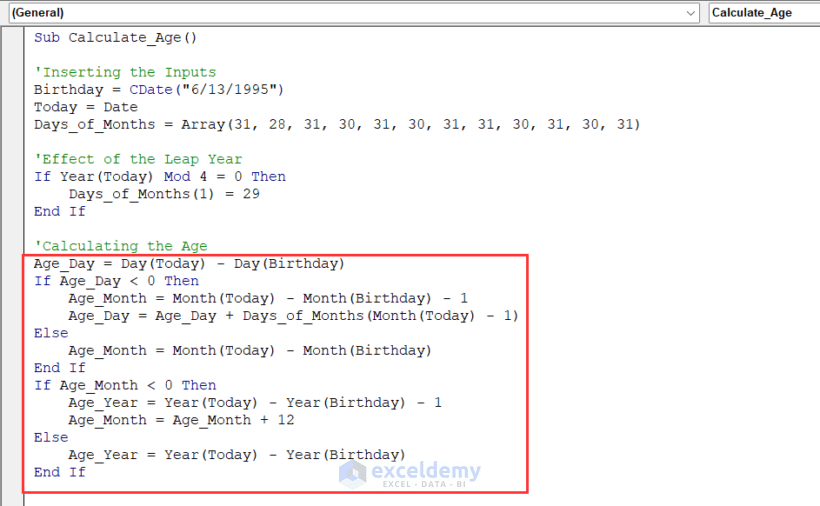# Excel VBA: Calculate Age from Date of Birth

Get FREE Advanced Excel Exercises with Solutions!

In this article, I’ll show you how you can calculate age from the date of birth using VBA in Excel.

VBA Code to Calculate Age from Date of Birth (Quick View)

``````Sub Calculate_Age()

'Inserting the Inputs
Birthday = CDate("6/13/1995")
Today = Date
Days_of_Months = Array(31, 28, 31, 30, 31, 30, 31, 31, 30, 31, 30, 31)

'Effect of the Leap Year
If Year(Today) Mod 4 = 0 Then
Days_of_Months(1) = 29
End If

'Calculating the Age
Age_Day = Day(Today) - Day(Birthday)
If Age_Day < 0 Then
Age_Month = Month(Today) - Month(Birthday) - 1
Age_Day = Age_Day + Days_of_Months(Month(Today) - 1)
Else
Age_Month = Month(Today) - Month(Birthday)
End If
If Age_Month < 0 Then
Age_Year = Year(Today) - Year(Birthday) - 1
Age_Month = Age_Month + 12
Else
Age_Year = Year(Today) - Year(Birthday)
End If
'Displaying the Age
MsgBox Str(Age_Year) + " Years, " + Str(Age_Month) + " Months, " + Str(Age_Day) + " Days."

End Sub``````## An Overview of the VBA Code to Calculate Age from Date of Birth in Excel (Step-by-Step Analysis)

So, without further delay, let’s go to our main discussion today. We’ll break down the VBA code step-by-step to learn how to calculate age from the date of birth using VBA.

⧪ Step 1: Inserting the Necessary Inputs

At the very outset of the code, we have to insert the necessary inputs into the code. The inputs include:

• The Date of Birth (Put your date of birth)
• Today’s Date (The VBA Date function returns this)
• An Array Containing the Number of Days of Each Month
``````'Inserting the Inputs
Birthday = CDate("6/13/1995")
Today = Date
Days_of_Months = Array(31, 28, 31, 30, 31, 30, 31, 31, 30, 31, 30, 31)``````⧪ Step 2: Effect of the Leap Year

Next, we’ve to put the effect of the leap year. If the current year is a leap year, the total number of days of February will be 29 in place of 28.

``````'Effect of the Leap Year
If Year(Today) Mod 4 = 0 Then
Days_of_Months(1) = 29
End If``````⧪ Step 3: Calculating the Age

This is the most important step. We’ll calculate the age from the birth date in this step. The calculation is a bit complex. I’m not showing it in detail.

``````'Calculating the Age
Age_Day = Day(Today) - Day(Birthday)
If Age_Day < 0 Then
Age_Month = Month(Today) - Month(Birthday) - 1
Age_Day = Age_Day + Days_of_Months(Month(Today) - 1)
Else
Age_Month = Month(Today) - Month(Birthday)
End If
If Age_Month < 0 Then
Age_Year = Year(Today) - Year(Birthday) - 1
Age_Month = Age_Month + 12
Else
Age_Year = Year(Today) - Year(Birthday)
End If
``````

⧪ Step 4: Displaying the Age

Finally, it’s time to display the age. You can change it according to your needs.

``````'Displaying the Age
MsgBox Str(Age_Year) + " Years, " + Str(Age_Month) + " Months, " + Str(Age_Day) + " Days."``````So the complete VBA code will be:

VBA Code:

``````Sub Calculate_Age()

'Inserting the Inputs
Birthday = CDate("6/13/1995")
Today = Date
Days_of_Months = Array(31, 28, 31, 30, 31, 30, 31, 31, 30, 31, 30, 31)

'Effect of the Leap Year
If Year(Today) Mod 4 = 0 Then
Days_of_Months(1) = 29
End If

'Calculating the Age
Age_Day = Day(Today) - Day(Birthday)
If Age_Day < 0 Then
Age_Month = Month(Today) - Month(Birthday) - 1
Age_Day = Age_Day + Days_of_Months(Month(Today) - 1)
Else
Age_Month = Month(Today) - Month(Birthday)
End If
If Age_Month < 0 Then
Age_Year = Year(Today) - Year(Birthday) - 1
Age_Month = Age_Month + 12
Else
Age_Year = Year(Today) - Year(Birthday)
End If

'Displaying the Age
MsgBox Str(Age_Year) + " Years, " + Str(Age_Month) + " Months, " + Str(Age_Day) + " Days."

End Sub``````Read More: How to Calculate Current Age in Excel

## Developing the Macro to Calculate Age from Date of Birth

We’ve seen the step-by-step analysis of the code to calculate age from the date of birth.

Now let’s see how to develop a macro to run the code.

⧪ Step 1: Opening VBA Window

Press ALT + F11 on your keyboard to open the Visual Basic window.⧪ Step 2: Inserting a New Module

Go to Insert > Module in the toolbar. Click on Module. A new module called Module1 (or anything else depending on your past history) will open.⧪ Step 3: Putting the VBA Code

This is the most important step. Insert the given VBA code in the module.⧪ Step 4: Running the Code

Click on the Run Sub / UserForm tool from the toolbar above.The code will run displaying the age in a Message Box.## Conclusion

Therefore, this is the process to calculate age from the date of birth using VBA in Excel. Do you have any questions? Feel free to ask us.

## What is ExcelDemy?

ExcelDemy Learn Excel & Excel Solutions Center provides free Excel tutorials, free support , online Excel training and Excel consultancy services for Excel professionals and businesses. Feel free to contact us with your Excel problems.Rifat Hassan

Hello! Welcome to my profile. Here I will be posting articles related to Microsoft Excel. I am a passionate Electrical Engineer holding a Bachelor’s degree in Electrical and Electronic Engineering from the Bangladesh University of Engineering and Technology. Besides academic studies, I always love to keep pace with the revolution in technology that the world is rushing towards day by day. I am diligent, career-oriented, and ready to cherish knowledge throughout my life.

1. ReplyThis is buggy, don’t use it. For compare against date of January the month – 2 will access an invalid position from the array and throw errors.

• ReplyTanjima Hossain Feb 15, 2023 at 3:23 PM

Hello Excel Guru,
Thanks for your comment. The article has been updated, so you can try the code now easily for January month also. And the previous code was not buggy obviously, it was used for another purpose and so it also gave results.
Thanks
ExcelDemyAdvanced Excel Exercises with Solutions PDF